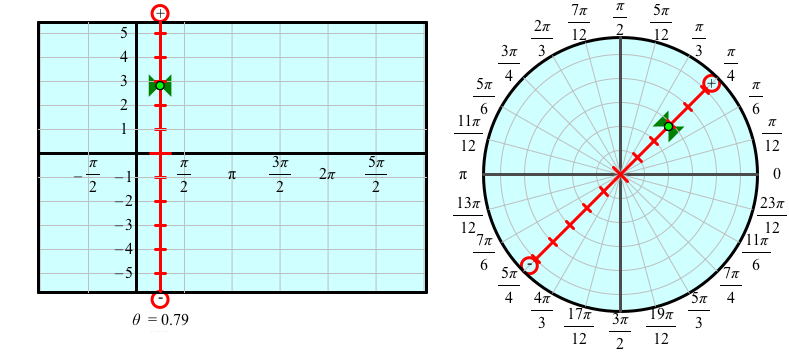# Cartesian and Polar Graphs

The May 2013 Mathematics Teacher has an excellent article by Jonathan F. Lawes (“Graphing Polar Curves”) on the value of plotting the same function in both polar and rectangular coordinates. Doing so not only helps students understand how polar coordinates work, but also gives them a novel and revealing perspective on periodic motion and how polar coordinates reveal periodicity.The sample activity Cartesian Graphs and Polar Graphs, shown above, comes with Sketchpad 5. After launching Sketchpad, choose Learning Center from the Help menu and navigate to “Trigonometry, Conics, and Precalculus” under Sample Activities. This activity and its accompanying sketch and worksheet provide a convenient and powerful way to engage students in exploring the relationships discussed in the article.

For each graph, the independent variable appears as a red bar that corresponds to a particular value of x (for Cartesian) or θ (for polar). The red bar has tick marks that show possible values of the dependent variable (y for Cartesian and r for polar), and a green bow-tie that shows the actual value of the dependent variable for this value of the independent variable. Here’s what it looks like for the function r = 4 cos θ when the slider for θ has been dragged to π/4:Remarkably, the two red bars (and their green bow-ties) are identical except that the Cartesian one has been translated from θ = 0 to θ = π/4, and the polar one has been rotated from θ = 0 to θ = π/4.

We designed both the red bar and the animation to connect the two representations as clearly as we could. After showing the graphs and turning on tracing, students can animate the independent variable. As the animation proceeds, students can observe how the two representations show exactly the same function, with the only difference being that the independent variable’s value undergoes translation on the left and rotation on the right.

There are many insights and discoveries students can make by dragging the independent variable and observing the graph. For instance, the seemingly counter-intuitive comparison between r = 4 cos 2θ on the left and r = 4 cos 3θ on the right becomes much clearer when students have the ability to control the value of θ by dragging. (Try it yourself first before reading the note at the end of the post.)

Other wonderful questions and realizations can come about by experimenting with a variety of functions. For instance, can you tell which of the polar graphs below represents the function r = tan θ and which represents r = 4 tan θ?

Here’s another fun challenge: Create the following polar graphs using simple trigonometric functions:

To create your own polar graphs from scratch, see my follow-up post.
This sketch implements Figures 3 through 9 from the article: Graphing Polar Curves Figures.gsp

Note: When you graph r = 4 cos 3θ by dragging θ from 0 to 2π, it’s really easy for students to see that the graph traces out six lobes, not three. The static graph shows only three, but as students drag they see how the six lobes overlap each other.

## 2 thoughts on “Cartesian and Polar Graphs”

1. Pingback: Polar Graphing |
2.Linda Kambadwa says:

The information being offered are very useful to us university students. Thank you.